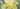# Implementing a Bloom filter - Part 2

Last time we talked about what a Bloom filter is and why you might use it. In this post we will cover how Bloom filters work internally and how they achieve constant time lookups.

We start off with an array of bits of a fixed size `n` (where `n` is dependent on the number of inserts we plan to make) which we initialize to zeroes. We will also need a list of `k` hashing functions. Each hashing function needs to map to an index into our array, so `0` -> `n-1`. It's important we pick uniformly distributed and different hashing functions, and we'll see in a minute why.

For this example we'll take `n = 10` and `k = 3`.

``````// Start off with our array of zeroes
[0, 0, 0, 0, 0, 0, 0, 0, 0, 0]``````

Now, let's see what putting an element does internally.

``````put("A")

// We take "A" and apply all k hashing functions to it
k1("A") -> 0
k2("A") -> 2
k3("A") -> 9

// Now we set all those bits to 1
// Note that we read the bits from the left, since we are
// talking about an array of single bits, not bits in a byte.
[1, 0, 1, 0, 0, 0, 0, 0, 0, 1]``````

Now let's do some queries.

First let's query for an element which is there. We expect true here.

``````isMaybePresent("A")

// We take "A" and apply all k hashing functions to it (again)
k1("A") -> 0
k2("A") -> 2
k3("A") -> 9

// Now we check those bits in our array.
// If we have at least one bit which is not set (0), we return false.
// If all bits are set (1), we return true.
// In this case, the bits are set, so we return true.
true``````

Now let's query for an element which we know is not there. We expect false here most of the time.

``````isMaybePresent("D")

// We take "D" and apply all k hashing functions to it
k1("D") -> 4
k2("D") -> 7
k3("D") -> 0

// In this case
// 4 is not set
// 7 is not set
// 0 is set (it was set by putting "A" in)
// So we have at least one bit which is not set, so we return false.
false``````

OK this makes sense but why can't we say for sure the element is there? When could we query for `"D"` without ever inserting it into the Bloom filter and get true? Let's put another element in.

``````put("B")

// We take "B" and apply all k hashing functions to it
k1("B") -> 7
k2("B") -> 3
k3("B") -> 4

// Now we set all those bits to 1
[1, 0, 1, 1, 1, 0, 0, 1, 0, 1]``````

Now let's query for `"D"` again.

``````isMaybePresent("D")

// We take "D" and apply all k hashing functions to it
k1("D") -> 4
k2("D") -> 7
k3("D") -> 0

// In this case
// 4 is set (it was set by putting "B" in)
// 7 is set (it was set by putting "B" in)
// 0 is set (it was set by putting "A" in)
// All the bits are now set, so we return true.
true``````

So the bits corresponding to hashing `"D"` were actually set by putting `"A"` and `"B"` in. This is why we got the false positive.

This example was deliberately constructed to produce this result, but in reality for a large bloom filter with more hashing functions, the probability of this hapenning is much lower (although it does happen). Tweaking `n` and `k` will produce different false positive rates.

So let's recap what the logic of the Bloom filter is.

Putting an element into the Bloom filter

• apply all k hashing functions to input
• set all bits defined by the hashing to 1

Checking whether an element is present in the Bloom filter

• apply all k hashing functions to input
• if all bits defined by the hashing are set (1) we return true, otherwise we return false

Next up, we'll look at how to implement this.